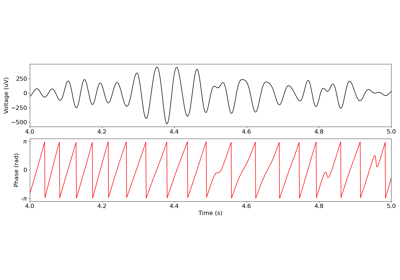# neurodsp.plts.plot_instantaneous_measure¶

neurodsp.plts.plot_instantaneous_measure(times, sigs, measure='phase', ax=None, **kwargs)[source]

Plot an instantaneous measure, of phase, amplitude or frequency.

Parameters
times1d array or list of 1d array

Time definition(s) for the time series to be plotted.

sigs1d array or list of 1d array

Time series to plot.

measure{‘phase’, ‘amplitude’, ‘frequency’}

Which kind of measure is being plotted.

axmatplotlib.Axes, optional

Figure axes upon which to plot.

**kwargs

Keyword arguments to pass into plot_time_series, and/or for customizing the plot.

Examples

Create an instantaneous phase plot:

>>> from neurodsp.sim import sim_combined
>>> from neurodsp.utils import create_times
>>> from neurodsp.timefrequency import phase_by_time
>>> sig = sim_combined(n_seconds=2, fs=500,
...                    components={'sim_powerlaw': {}, 'sim_oscillation' : {'freq': 10}})
>>> pha = phase_by_time(sig, fs=500, f_range=(8, 12))
>>> times = create_times(n_seconds=2, fs=500)
>>> plot_instantaneous_measure(times, pha, measure='phase')


## Examples using neurodsp.plts.plot_instantaneous_measure¶